# 高级线性表——静态链表(最全静态链表解读)

1.静态链表的概述
2.静态链表的实现

3.静态链表的代码实现

4.静态链表的完整代码实现及演示
5.静态链表和动态链表的联系和区别

# 1.静态链表的概述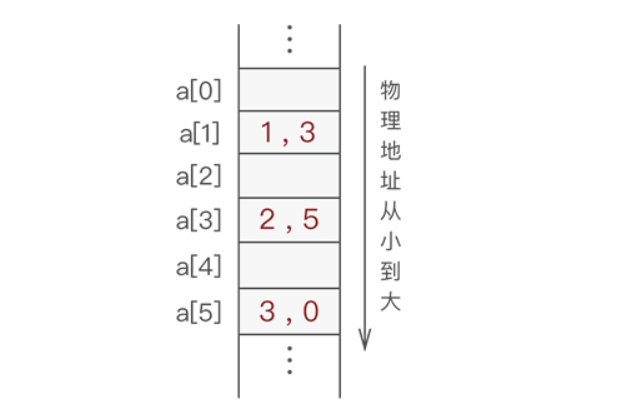`如上通过 "数组+游标" 的方式存储具有线性关系数据的存储结构就是静态链表。`

# 2.静态链表的实现

#### 2.1静态链表的结点

1. 数据域：用于存储数据元素的值；
2. 游标：其实就是数组下标，表示直接后继元素所在数组中的位置；

``typedef struct {     int data;//数据域     int cur;//游标 }component; ``

#### 2.2备用链表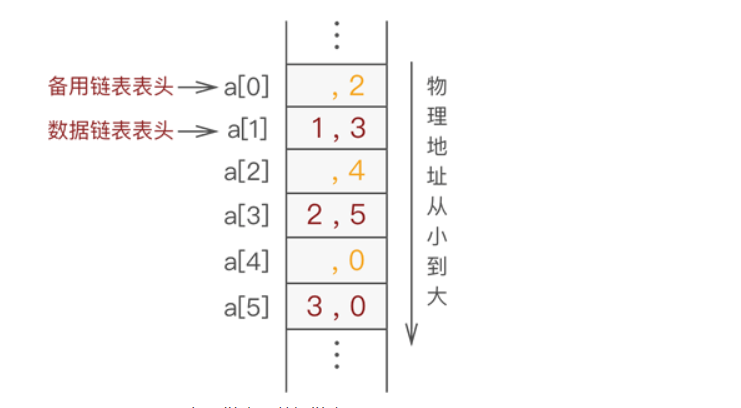上图备用链表上连接的依次是 a、a 和 a，而数据链表上连接的依次是 a、a 和 a。

#### 2.3静态链表的详细实现过程

1.在数据链表未初始化之前，数组中所有位置都处于空闲状态，因此都应被链接在备用链表上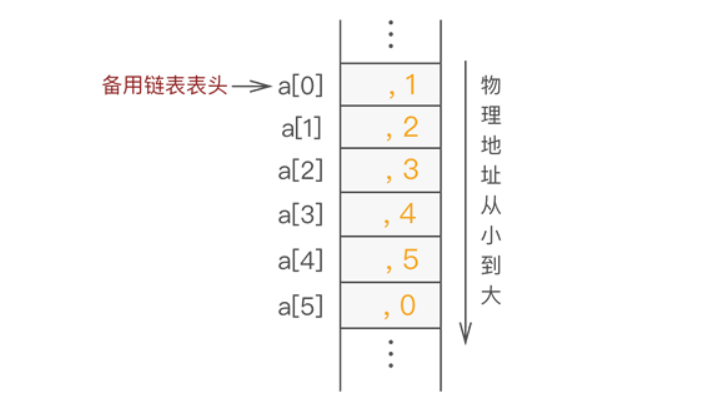2 .因此，在上图的基础上，向静态链表中添加元素 1 的过程下图 所示：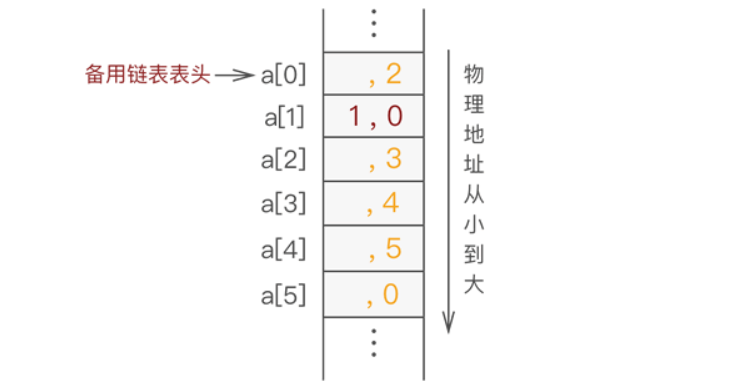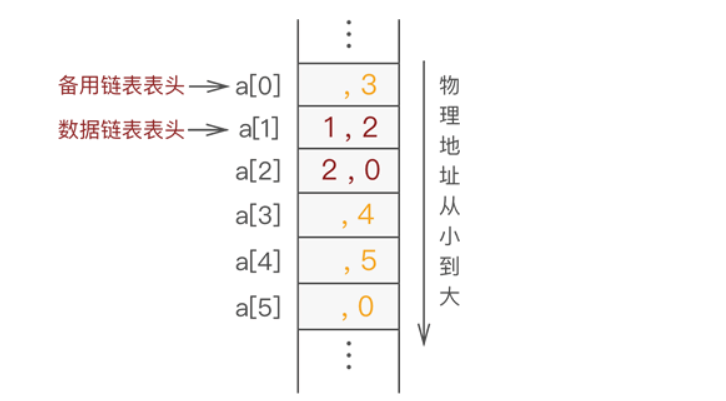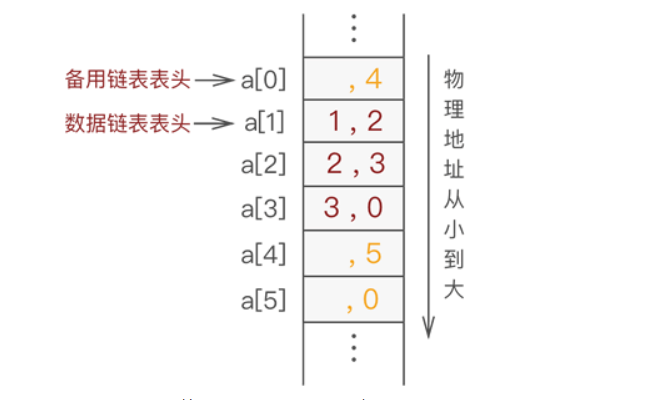# 3.静态链表的代码实现(最后一个数组元素相当于头结点)

#### 3.1静态链表初始化

``void InitArr(component *array) {     int i = 0;     for (i = 0; i < maxSize; i++) {         array[i].cur = i + 1;//将每个数组分量链接到一起         array[i].data = 0;     }     array[maxSize - 1].cur = 0;//链表最后一个结点的游标值为0 } ``

#### 3.2静态链表的提取分配空间

``int mallocArr(component * array) {     //若备用链表非空，则返回分配的结点下标，否则返回 0（当分配最后一个结点时，     //该结点的游标值为 0）     int i = array.cur;     if (array.cur) {         array.cur = array[i].cur;     }     return i; } ``

#### 3.3静态链表添加元素

``//向链表中插入数据，body表示链表的头结点在数组中的位置，add表示插入元素的位置，num表示要插入的数据 void insertArr(component * array, int body, int add, int num) {     int tempBody = body;//tempBody做遍历结构体数组使用     int i = 0, insert = 0;     //找到要插入位置的上一个结点在数组中的位置     for (i = 1; i < add; i++) {         tempBody = array[tempBody].cur;     }     insert = mallocArr(array);//申请空间，准备插入     array[insert].data = num;      array[insert].cur = array[tempBody].cur;//新插入结点的游标等于其直接前驱结点的游标     array[tempBody].cur = insert;//直接前驱结点的游标等于新插入结点所在数组中的下标 } ``

#### 3.4释放空间

``void freeArr(component * array, int k) {     array[k].cur = array.cur;     array.cur = k; } ``

#### 3.5静态链表删除元素

``//删除结点函数，num表示被删除结点中数据域存放的数据 int deletArr(component * array, int num) {     int tempBody =maxSize-1;     int del = 0;     int newbody = 0;     //找到被删除结点的位置     while (array[tempBody].data != num) {         tempBody = array[tempBody].cur;         //当tempBody为0时，表示链表遍历结束，说明链表中没有存储该数据的结点         if (tempBody == 0) {             printf("链表中没有此数据");             return 0;         }     }     //运行到此，证明有该结点     del = tempBody;     tempBody = maxSize-1;     //删除首元结点，需要特殊考虑     if (del == array[maxSize-1].cur) {         newbody = array[del].cur;         array[maxSize-1].cur=newbody;          freeArr(array, del);     }     else     {         //找到该结点的上一个结点，做删除操作         while (array[tempBody].cur != del) {             tempBody = array[tempBody].cur;         }         //将被删除结点的游标直接给被删除结点的上一个结点         array[tempBody].cur = array[del].cur;         //回收被摘除节点的空间         freeArr(array, del);     }   } ``

#### 3.6修改元素

``void amendElem(component * array, int oldElem, int newElem) {     int add = selectNum(array,oldElem);     if (add == -1) {         printf("无更改元素");         return;     }     array[add].data = newElem; }  ``

#### 3.7查找元素

``int selectNum(component * array, int num) {     //当游标值为0时，表示链表结束      int tempBody = maxSize-1;      while (array[tempBody].cur != 0) {         if (array[tempBody].data == num) {             return tempBody;         }         tempBody = array[tempBody].cur;     }     //判断最后一个结点是否符合要求     if (array[tempBody].data == num) {         return tempBody;     }     return -1;//返回-1，表示在链表中没有找到该元素 } ``

# 4.静态链表完整代码实现及演示

``#include <stdio.h> #define maxSize 6 typedef struct {     int data;     int cur; }component; void InitArr(component *array) {     int i = 0;     for (i = 0; i < maxSize; i++) {         array[i].cur = i + 1;//将每个数组分量链接到一起         array[i].data = 0;     }     array[maxSize - 1].cur = 0;//链表最后一个结点的游标值为0 } int mallocArr(component * array) {     //若备用链表非空，则返回分配的结点下标，否则返回 0（当分配最后一个结点时，     //该结点的游标值为 0）     int i = array.cur;     if (array.cur) {         array.cur = array[i].cur;     }     return i; } //初始化几个元素  void add(component * array) { 	printf("请输入你想添加的数据成员的数量："); 	int n; 	scanf("%d",&n); 	for(int i=1;i<=n;i++) 	{ 		printf("请输入第%d个数据成员：",i); 		scanf("%d",&array[i].data);	 	} 	array.cur=n+1; 	array[n].cur=0; 	array[maxSize-1].cur=1; } //向链表中插入数据,add表示插入元素的位置，num表示要插入的数据 void insertArr(component * array, int add, int num) {     int tempBody = maxSize-1;//tempBody做遍历结构体数组使用     int i = 0, insert = 0;     //找到要插入位置的上一个结点在数组中的位置     for (i = 1; i < add; i++) {         tempBody = array[tempBody].cur;     }     insert = mallocArr(array);//申请空间，准备插入     array[insert].data = num;      array[insert].cur = array[tempBody].cur;//新插入结点的游标等于其直接前驱结点的游标     array[tempBody].cur = insert;//直接前驱结点的游标等于新插入结点所在数组中的下标 } void displayArr(component * array) {     int tempBody = maxSize-1;//tempBody准备做遍历使用     tempBody=array[tempBody].cur;     while (array[tempBody].cur) {         printf("%d,%d ", array[tempBody].data, array[tempBody].cur);         tempBody = array[tempBody].cur;     }     printf("%d,%dn", array[tempBody].data, array[tempBody].cur); } //查找数据域为elem的结点在数组中的位置 int selectNum(component * array, int num) {     //当游标值为0时，表示链表结束      int tempBody = maxSize-1;      while (array[tempBody].cur != 0) {         if (array[tempBody].data == num) {             return tempBody;         }         tempBody = array[tempBody].cur;     }     //判断最后一个结点是否符合要求     if (array[tempBody].data == num) {         return tempBody;     }     return -1;//返回-1，表示在链表中没有找到该元素 }  //在以body作为头结点的链表中将数据域为oldElem的结点，数据域改为newElem void amendElem(component * array, int oldElem, int newElem) {     int add = selectNum(array,oldElem);     if (add == -1) {         printf("无更改元素");         return;     }     array[add].data = newElem; }   void freeArr(component * array, int k) {     array[k].cur = array.cur;     array.cur = k; }  //删除结点函数，num表示被删除结点中数据域存放的数据，函数返回新数据链表的表头位置 int deletArr(component * array, int num) {     int tempBody =maxSize-1;     int del = 0;     int newbody = 0;     //找到被删除结点的位置     while (array[tempBody].data != num) {         tempBody = array[tempBody].cur;         //当tempBody为0时，表示链表遍历结束，说明链表中没有存储该数据的结点         if (tempBody == 0) {             printf("链表中没有此数据");             return 0;         }     }     //运行到此，证明有该结点     del = tempBody;     tempBody = maxSize-1;     //删除首元结点，需要特殊考虑     if (del == array[maxSize-1].cur) {         newbody = array[del].cur;         array[maxSize-1].cur=newbody;          freeArr(array, del);     }     else     {         //找到该结点的上一个结点，做删除操作         while (array[tempBody].cur != del) {             tempBody = array[tempBody].cur;         }         //将被删除结点的游标直接给被删除结点的上一个结点         array[tempBody].cur = array[del].cur;         //回收被摘除节点的空间         freeArr(array, del);     }   } int main() { 	component array[maxSize];     InitArr(array);     add(array);     int selectAdd;     printf("静态链表为：n");     displayArr(array);     printf("在第3的位置上插入元素4:n");     insertArr(array, 3, 4);     displayArr(array);     printf("删除数据域为1的结点:n");     deletArr(array, 1);     displayArr(array);     printf("查找数据域为4的结点的位置:n");     selectAdd = selectNum(array, 4);     printf("%dn", selectAdd);     printf("将结点数据域为4改为5:n");     amendElem(array, 4, 5);     displayArr(array);     return 0;  }  ``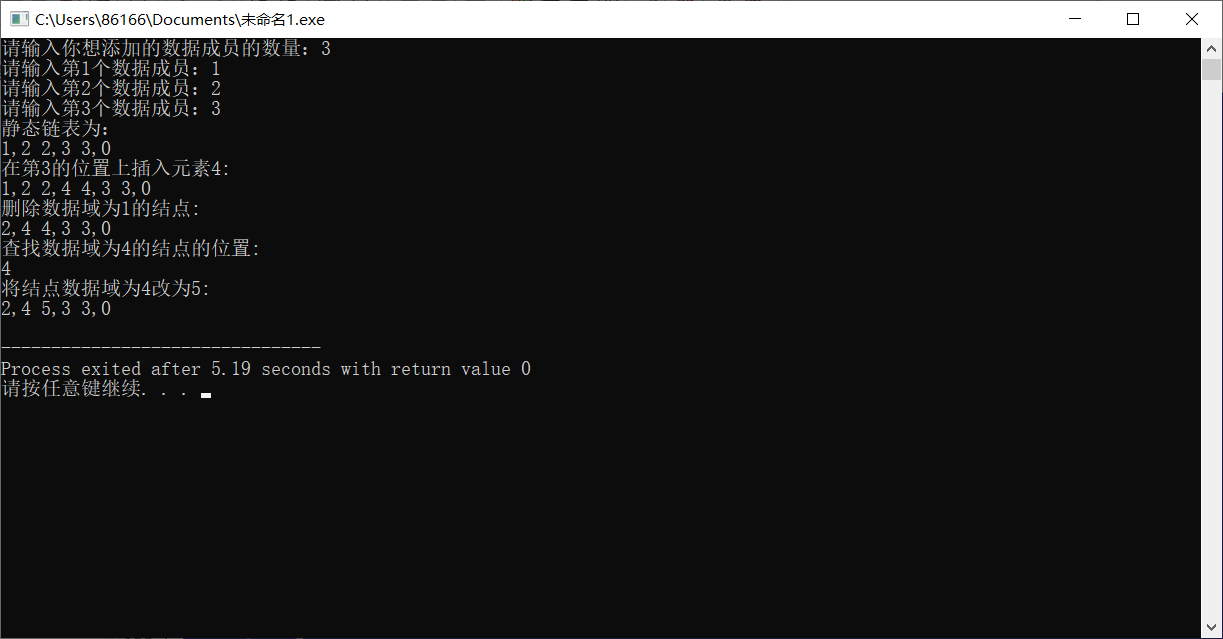# 静态链表和动态链表的联系和区别

#### 5.2动态链表(普通链表)

qklbishe.com区块链毕设代做网专注|以太坊fabric-计算机|java|毕业设计|代做平台 » 高级线性表——静态链表(最全静态链表解读)